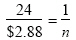Name:    3rd Grade Math Proficient  Test 10

Multiple Choice
Identify the choice that best completes the statement or answers the question.

1.

Problem Solving – RIT 201 – 210

If there are 10 slices in a pizza, and 23 children want to order enough pizzas
so they can each have 2 slices, about how many pizzas should they order?
 a. 5 d. 3 b. 23 e. 2 c. 10

2.

Trina went to the mall. She bought a sweater for \$15.00, a pair of shoes
for \$25.00, and an ice cream cone for \$2.00. How much money did she
spend?
 a. \$32.00 d. \$47.00 b. \$42.00 e. \$52.00 c. \$37.00

3.

What are the prime numbers between 20 and 30?
 a. 27, 29 d. 23, 27 b. 21, 23 e. 21, 29 c. 29, 23

4.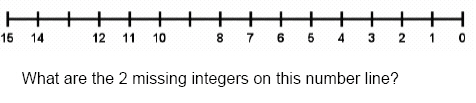a. 16, 9, 1 d. 13, 9 b. 16, 13 e. 8, 12 c. 1, 9

5.a. 40% d. 43% b. 30% e. 70% c. 33%

6.

Johnny’s shoelaces are 1 foot long. Suzie’s shoelaces are 8 inches long.
How much longer are Johnny’s shoelaces than Suzie’s?
 a. 2 inches d. 9 inches b. 4 inches e. 12 inches c. 6 inches

7.

If a car is traveling 60 miles per hour and does not change speed or make any
stops, how long will it take to go 300 miles?
 a. 5 hours d. 7 hours b. 6 hours e. 3 hours c. 4 hours

8.

John has 4 coins totallying 85. He has only quarters and dimes. How many
dimes does John have?
 a. 3 d. 6 b. 2 e. 5 c. 1

9.

If it rains 2 inches every 30 days, how much rain would fall in 90 days?
 a. 6 inches d. 45 inches b. 30 inches e. 3 inches c. 15 inches

10.

Which figure has parallel lines?
 a.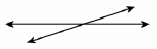d.b.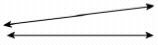e.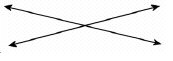c.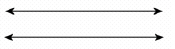11.

40 candy canes come in a box. 10 candy canes were broken. How many
candy canes were not broken?
How would you find the answer?
 a. addition c. multiplication b. subtraction d. division

12.

Billy starts soccer at 2:45 P.M. He practices for 35 minutes. What time
does practice end?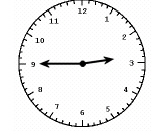a. 3:15 d. 3:20 P.M. b. 4:00 P.M. e. 3:30 A.M. c. 3:15 P.M.

13.

What is the probability that the spinner will land on yellow?a. 2 out of 4 d. 1 out of 4 b. 1 out of 6 e. 4 out of 6 c. 2 out of 6

14.

The Wongs are going to fence their yard. How many feet of fence do they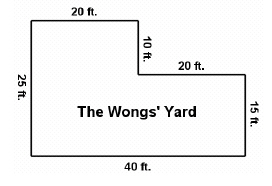a. 100 feet d. 120 feet b. 110 feet e. 130 feet c. 65 feet

15.

9, 767, 422, 135
What is the value of the digit in the one billions place?
 a. 9 d. 9,000 b. 9,000,000,000 e. 900 c. 9,000,000

16.

Diana received scores of 100, 63, 90, 85, and 92 on her math tests. What
is her mean (average) score?
 a. 81 d. 415 b. 82 e. 85 c. 83

17.

There are 60 marbles in a jar. If 8 children get the same number of
marbles, how many will be left over?
 a. 52 d. 4 b. 8 e. 0 c. 11

18.

A jacket costs \$25. It is on sale for a 20% discount. How much is the sale
price?
 a. \$5.00 d. \$20.00 b. \$30.00 e. \$45.00 c. \$50.00

19.

Betty’s Bakery sells chocolate chip, oatmeal, and peanut butter cookies.
They come in two sizes, regular and jumbo. What are the possible
combinations you can get if you buy 2 boxes of cookies?
 a. regular chocolate chip, regular peanut butter, regular oatmeal b. regular chocolate chip, regular peanut butter, jumbo chocolate chip,jumbo peanut butter c. jumbo chocolate chip, jumbo peanut butter, jumbo oatmeal d. regular chocolate chip, regular peanut butter, regular oatmeal,jumbo chocolate chip, jumbo peanut butter, jumbo oatmeal e. oatmeal, chocolate chip, peanut butter

20.

If you have two tables that seat 6 people each, how many people can you
seat if you push the two tables together?a. 12 d. 11 b. 8 e. 10 c. 6

21.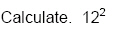a. 14 d. 144 b. 10 e. 5 c. 24

22.

Use proportions to solve the following problem.
If a package of 24 pencils costs \$2.88, how much will 1 pencil cost?

 a.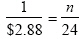d.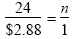b.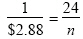e.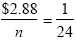c.